Question

# A machine set to fill soup cans with a mean of 20 ounces and a standard...

A machine set to fill soup cans with a mean of 20 ounces and a standard deviation of 0.1 ounces. A random sample of 34 cans has a mean of 20.02 ounces. Should the machine be reset?

No, the probability of this outcome at 0.122, would be considered usual, so there is no problem

No the probability of this outcome at 0.421 would be considered usual, so there is no problem

Yes, the probability of this outcome at 0.878 would be considered unusual, so the machine should be reset

Yes, the probability of this outcome at 0.122, would be considered unusual, so the machine should be reset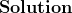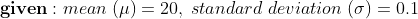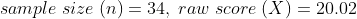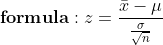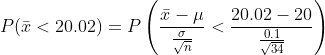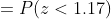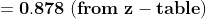Yes, the probability of this outcome at 0.878 would be considered unusual, so the machine should be reset

#### Earn Coins

Coins can be redeemed for fabulous gifts.

Similar Homework Help Questions
• ### A machine used to fill gallon-sized paint cans is regulated so that the amount of paint...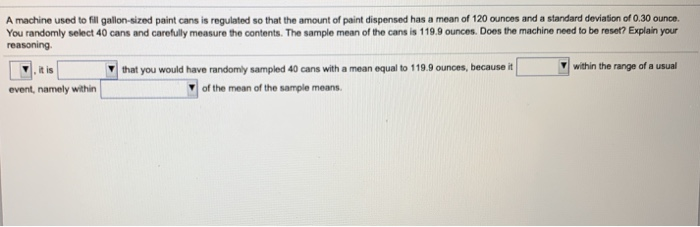A machine used to fill gallon-sized paint cans is regulated so that the amount of paint dispensed has a mean of 120 ounces and a standard deviation of 0.30 ounce You randomly select 40 cans and carefully measure the contents. The sample mean of the cans is 119.9 ounces. Does the machine need to be reset? Explain your reasoning it is that you would have randomly sampled 40 cans with a mean equal to 119.9 ounces, because it within the...

• ### Soft drink cans are filled by an automated filling machine and the standard deviation is 0.5...

Soft drink cans are filled by an automated filling machine and the standard deviation is 0.5 fluid ounce. Assume that the fill volumes of the cans are independent, normal random variables. Round your answers to four decimal places (e.g. 98.7654). What is the standard deviation of the average fill volume of 100 cans? If the mean fill volume 12.1 ounces, what is the probability that the average fill volume of the 100 cans is less than 12 fluid ounces? What...

• ### A machine used to fill cans of Campbell’s tomato soup (low salt) has the following characteristics:...

A machine used to fill cans of Campbell’s tomato soup (low salt) has the following characteristics: µ = 12 ounces and s = .5 ounces. a. Depict graphically the sampling distribution of all possible values of , where is the sample mean (point estimator) for 30 cans selected randomly by a quality control inspector. b. What is the probability of selecting a sample of 36 cans with a sample mean greater than 12.2 ounces?

• ### Soft drink cans are filled by an automated filling machine and the standard deviation is 0.5...

Soft drink cans are filled by an automated filling machine and the standard deviation is 0.5 fluid ounce. Assume that the fill volumes of the cans are independent, normal random variables. Round your answers to four decimal places (e.g. 98.7654). If the mean fill volume is 12.1 fluid ounces, what should the standard deviation of fill volume equal so that the probability that the average of 100 cans is less than 12 fluid ounces is 0.005?

• ### The fill volume of an automated filling machine used for filling cans of carbonated beverage is...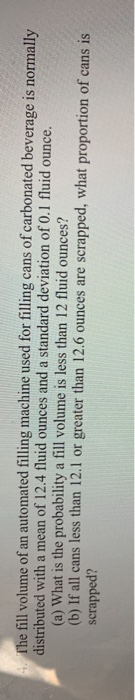The fill volume of an automated filling machine used for filling cans of carbonated beverage is normally distributed with a mean of 12.4 fluid Ounces and a standard deviation of 0.1 fluid Ounce. (a) What is the probability a fill volume is less than 12 fluid Ounces? (b) If all cans less than 12.1 or greater than 12.6 ounces are scrapped, what proportion of cans is scrapped?

• ### Sampling Distributions 1. An automatic machine used to ill cans of soup has a mean flling...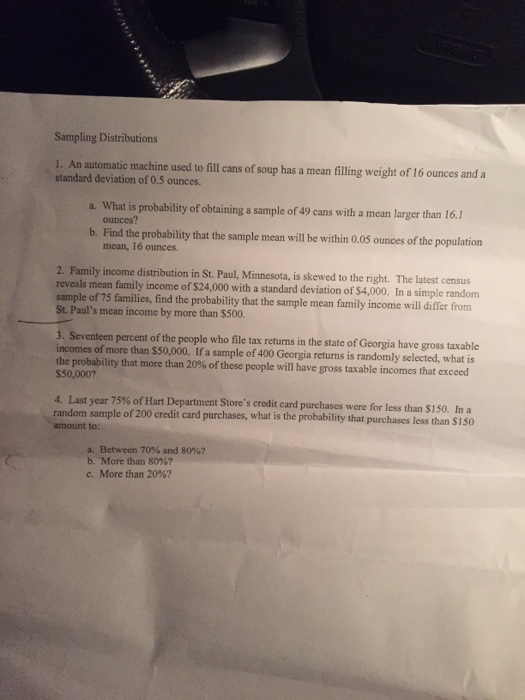Sampling Distributions 1. An automatic machine used to ill cans of soup has a mean flling weight of 16 ounces and a standard deviation of 0.5 ounces. a. What is probability of obtaining a sample of 49 cans with a mean larger than 16.1 b. Find the probability that the sample mean will be within 005 ounces of the population ounces? mean, 16 ounces. 2. Family income distribution in St. Paul, Minnesota, is skewed to the right. The latest census...

• ### the amount of liquid in cans of a cola beverage has mean value 16 ounces and...

the amount of liquid in cans of a cola beverage has mean value 16 ounces and standard deviation of 0.143 ounces (a) what is the probability that a randomly selected can of that cola beverage contains at least 15.9 ounces? (b) what is the probability that the mean amount x of beverage in a random sample of 34 such cans is at least 16.1 ounces

• ### The coffee and soup machine at the local subway station is supposed to fill cups with...

The coffee and soup machine at the local subway station is supposed to fill cups with 6 ounces of soup. Ten cups of soup are brought with results of a mean of 5.93 ounces and a standard deviation of 0.13 ounces. How large a sample of soups would we need to be 95 percent confident that the sample mean is within 0.03 ounces of the population mean? 97 96 73 62 10

• ### 3. The fill volume of an automated filling machine used for filling cans of carbonated beverage...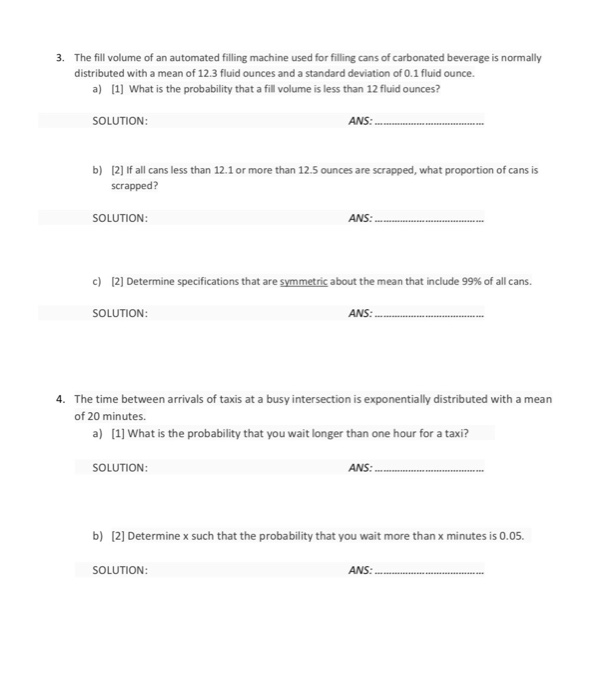3. The fill volume of an automated filling machine used for filling cans of carbonated beverage is normally distributed with a mean of 12.3 fluid ounces and a standard deviation of 0.1 fluid ounce. a) 1] What is the probability that a fill volume is less than 12 fluid ounces? SOLUTION ANS: b) 2] If all cans less than 12.1 or more than 12.5 ounces are scrapped, what proportion of cans is scrapped? SOLUTION: ANS: c)  Determine specifications that...

• ### core: 0 of 2 pts .4.35 A machine used to fil galon-sized paint cans is reguiated...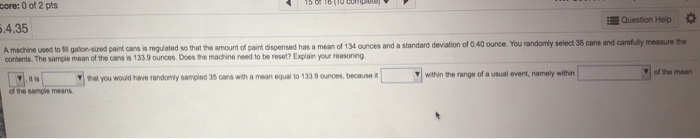core: 0 of 2 pts .4.35 A machine used to fil galon-sized paint cans is reguiated Question Hoip so that the amount of paint dispensed has a mean of 134 ounces and a standard deviation of 0.40 ounce. You randomly select 35 cans and carefully measure the contents.The sample mean of the cans is 1339 ounces. Does the machine need to be reset? Explain your reasoning that you would have randomly sampled 35 cans with a mean equal to 1330ounoes,...